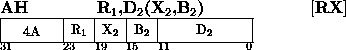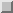The second operand is added to the first operand, and the sum is placed in the first-operand location, The second operand is two bytes in length and is considered to be a 16-bit signed integer.

The second operand is expanded to 32 bits before the addition by propagating the sign-bit value through the 16 high-order bit positions. The contents of the second operand in main storage remain unchanged.

Addition is performed by adding all 32 bits of both operands. If the carry out of the sign-bit position and the carry out of the high-order numeric bit position agree, the sum is satisfactory; if they disagree, an overflow occurs. The sign bit is not changed after the overflow. A positive overflow yields a negative final sum, and a negative overflow results in a positive sum. The overflow causes a program interruption when the fixed-point overflow mask bit is one.

Resulting Condition Code:
0 ~ Sum is zero
1 ~ Sum is less than zero
2 ~ Sum is greater than zero
3 ~ Overflow

Program Exceptions:
Access (fetch, operand 2)
Fixed-Point Overflow
Programming Note
See Extended Mnemonic Instructions on page